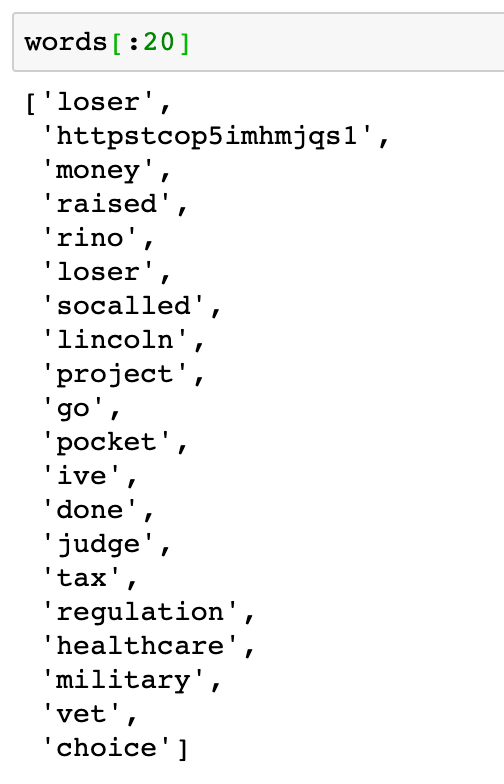# From DataFrame to N-Grams

A quick-start guide to creating and visualizing n-gram ranking using nltk for natural language processing.

When I was first starting to learn NLP, I remember getting frustrated or intimidated by information overload so I’ve decided to write a post that covers the bare minimum. You know what they say, “Walk before you run!”

This is a very gentle introduction so we won’t be using any fancy code here.

In a nutshell, natural language processing or NLP simply refers to the process of reading and understanding written or spoken language using a computer. At its simplest use case, we can use a computer to read a book, for example, and count how many times each word was used instead of us manually doing it.

NLP is a big topic and there’s already been a ton of articles written on the subject so we won’t be covering that here. Instead, we’ll focus on how to quickly do one of the simplest but useful techniques in NLP: N-gram ranking.

# N-Gram Ranking

Simply put, an n-gram is a sequence of n words where n is a discrete number that can range from 1 to infinity! For example, the word “cheese” is a 1-gram (unigram). The combination of the words “cheese flavored” is a 2-gram (bigram). Similarly, “cheese flavored snack” is a 3-gram (trigram). And “ultimate cheese flavored snack” is a 4-gram (qualgram). So on and so forth.

In n-gram ranking, we simply rank the n-grams according to how many times they appear in a body of text — be it a book, a collection of tweets, or reviews left by customers of your company.

Let’s get started!

# Getting the Data

First, let’s get our data and load it into a dataframe. You can download the sample dataset here or create your own from the Trump Twitter Archive.

`import pandas as pddf = pd.read_csv('tweets.csv')`

Using `df.head()` we can quickly get acquainted with the dataset.A sample of President Trump’s tweets.

# Importing Packages

Next, we’ll import packages so we can properly set up our Jupyter notebook:

`# natural language processing: n-gram rankingimport reimport unicodedataimport nltkfrom nltk.corpus import stopwords# add appropriate words that will be ignored in the analysisADDITIONAL_STOPWORDS = ['covfefe']import matplotlib.pyplot as plt`

In the code block above, we imported pandas so that we can shape and manipulate our data in all sorts of different and wonderful ways! Next, we imported `re` for regex, `unicodedata` for Unicode data, and `nltk` to help with parsing the text and cleaning them up a bit. And then, we specified additional stop words that we want to ignore. This is helpful in trimming down the noise. Lastly, we imported `matplotlib` matplotlib so we can visualize the result of our n-gram ranking later.

Next, let’s create a function that will perform basic cleaning of the data.

# Basic Cleaning

`def basic_clean(text):  """  A simple function to clean up the data. All the words that  are not designated as a stop word is then lemmatized after  encoding and basic regex parsing are performed.  """  wnl = nltk.stem.WordNetLemmatizer()  stopwords = nltk.corpus.stopwords.words('english') + ADDITIONAL_STOPWORDS  text = (unicodedata.normalize('NFKD', text)    .encode('ascii', 'ignore')    .decode('utf-8', 'ignore')    .lower())  words = re.sub(r'[^\w\s]', '', text).split()  return [wnl.lemmatize(word) for word in words if word not in stopwords]`

The function above takes in a list of words or text as input and returns a cleaner set of words. The function does normalization, encoding/decoding, lower casing, and lemmatization.

Let’s use it!

`words = basic_clean(''.join(str(df['text'].tolist())))`

Above, we’re simply calling the function `basic_lean()` to process the `'text'` column of our dataframe `df` and making it a simple list with `tolist()`. We then assign the results to `words`.A list of already cleaned, normalized, and lemmatized words.

# N-grams

Here comes the fun part! In one line of code, we can find out which bigrams occur the most in this particular sample of tweets.

`(pd.Series(nltk.ngrams(words, 2)).value_counts())[:10]`

We can easily replace the number 2 with 3 so we can get the top 10 trigrams instead.

`(pd.Series(nltk.ngrams(words, 3)).value_counts())[:10]`

Voilà! We got ourselves a great start. But why stop now? Let’s try it and make a little eye candy.

# Bonus Round: Visualization

To make things a little easier for ourselves, let’s assign the result of n-grams to variables with meaningful names:

`bigrams_series = (pd.Series(nltk.ngrams(words, 2)).value_counts())[:12]trigrams_series = (pd.Series(nltk.ngrams(words, 3)).value_counts())[:12]`

I’ve replaced `[:10]` with `[:12]` because I wanted more n-grams in the results. This is an arbitrary value so you can choose whatever makes the most sense to you according to your situation.

Let’s create a horizontal bar graph:

`bigrams_series.sort_values().plot.barh(color='blue', width=.9, figsize=(12, 8))`

And let’s spiffy it up a bit by adding titles and axis labels:

`bigrams_series.sort_values().plot.barh(color='blue', width=.9, figsize=(12, 8))plt.title('20 Most Frequently Occuring Bigrams')plt.ylabel('Bigram')plt.xlabel('# of Occurances')`

And that’s it! With a few simple lines of code, we quickly made a ranking of n-grams from a Pandas dataframe and even made a horizontal bar graph out of it.

I hope you enjoyed this one. Natural Language Processing is a big topic but I hope that this gentle introduction will encourage you to explore more and expand your repertoire.

In the next article, we’ll visualize an n-gram ranking in Power BI with a few simple clicks of the mouse and a dash of Python!

Stay tuned!

You can reach me on Twitter or LinkedIn.

This article was first published on Towards Data Science‘ Medium publication.